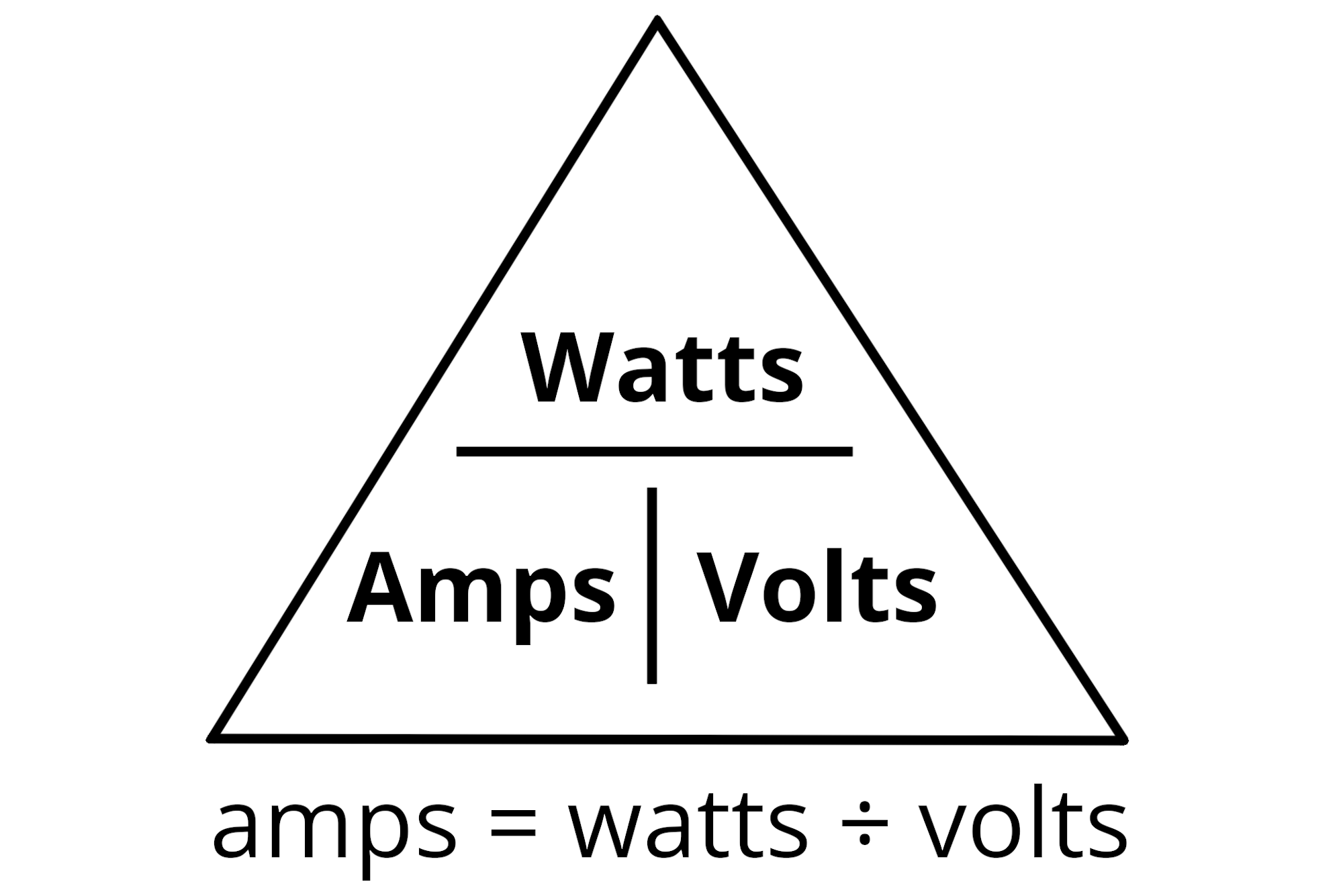# Watts to Amps Electrical Conversion Calculator

Enter the power and voltage to convert watts to amps for DC, single-phase AC, and three-phase AC circuits.

## Results:

amps
milliamps

Try our amps to watts calculator.

## How to Convert Watts to Amps

Converting watts to amps can be done using the power formula, which states that I = P ÷ E, where P is power measured in watts, I is current measured in amps, and E is voltage measured in volts.

Given this, to find amps given power and voltage use the following formula:

I(A) = P(W)V(V)

Thus, the current I in amps is equal to the power P in watts divided by the voltage V in volts.For example, find the amperage of 1200 watts at 120 volts

current = power ÷ voltage
current = 1200W ÷ 120V
current = 10A

## Single-Phase AC Circuit Watts to Amps Conversion

Converting watts to amps for a single-phase AC circuit with power factor uses a slightly different formula.

I(A) = P(W)V(V) × PF

In other words, the current I in amps is equal to the power P in watts divided by the voltage V in volts multiplied by the power factor PF. If you’re unsure what the power factor is then a power factor calculator can help.

## Three-Phase AC Circuit Watts to Amps Conversion

### Using Line to Line Voltage

For three-phase AC circuits where the line to line voltage is known, the formula to convert watts to amps is:

I(A) = P(W)VL-L(V) × PF × √3

The current I in amps is equal to the power P in watts divided by the line to line voltage V in volts multiplied by the power factor PF multiplied by the square root of 3.

### Using Line to Neutral Voltage

For three-phase AC circuits where the line to neutral voltage is known, the formula to convert watts to amps is:

I(A) = P(W)VL-N(V) × PF × 3

The current I in amps is equal to the power P in watts divided by the voltage V in volts multiplied by the power factor PF multiplied by 3.

## How to Convert Watts and Ohms to Amps

It is also possible to convert watts to amps if the resistance of the circuit is known using the formula:

I(A) = √(P(W) × R(Ω))

The current I in amps is equal to the square root of the power P in watts multiplied by the resistance R in ohms.

It is not possible to convert watts directly to amps without also knowing voltage or resistance.

Because 1 kilowatt is equal to 1,000 watts, it is possible to use the formulas above to also convert kW to amps, but watts need to be converted to kW first. Use our kW to amps calculator to solve for kilowatts.

## Equivalent Watts and Amps at 120V AC

wattage to amperage conversions at 120 volts.
Power Current Voltage
50 Watts 0.4167 Amps 120 Volts
100 Watts 0.8333 Amps 120 Volts
150 Watts 1.25 Amps 120 Volts
200 Watts 1.667 Amps 120 Volts
250 Watts 2.083 Amps 120 Volts
300 Watts 2.5 Amps 120 Volts
350 Watts 2.917 Amps 120 Volts
400 Watts 3.333 Amps 120 Volts
450 Watts 3.75 Amps 120 Volts
500 Watts 4.167 Amps 120 Volts
600 Watts 5 Amps 120 Volts
700 Watts 5.833 Amps 120 Volts
800 Watts 6.667 Amps 120 Volts
900 Watts 7.5 Amps 120 Volts
1000 Watts 8.333 Amps 120 Volts
1100 Watts 9.167 Amps 120 Volts
1200 Watts 10 Amps 120 Volts
1300 Watts 10.833 Amps 120 Volts
1400 Watts 11.667 Amps 120 Volts
1500 Watts 12.5 Amps 120 Volts
1600 Watts 13.333 Amps 120 Volts
1700 Watts 14.167 Amps 120 Volts
1800 Watts 15 Amps 120 Volts
1900 Watts 15.833 Amps 120 Volts
2000 Watts 16.667 Amps 120 Volts
2100 Watts 17.5 Amps 120 Volts
2200 Watts 18.333 Amps 120 Volts
2300 Watts 19.167 Amps 120 Volts
2400 Watts 20 Amps 120 Volts
2500 Watts 20.833 Amps 120 Volts

## Equivalent Watts and Amps at 12V DC

Equivalent wattage and amperage values at 12 volts.
Power Current Voltage
5 Watts 0.4167 Amps 12 Volts
10 Watts 0.8333 Amps 12 Volts
15 Watts 1.25 Amps 12 Volts
20 Watts 1.667 Amps 12 Volts
25 Watts 2.083 Amps 12 Volts
30 Watts 2.5 Amps 12 Volts
35 Watts 2.917 Amps 12 Volts
40 Watts 3.333 Amps 12 Volts
45 Watts 3.75 Amps 12 Volts
50 Watts 4.167 Amps 12 Volts
60 Watts 5 Amps 12 Volts
70 Watts 5.833 Amps 12 Volts
80 Watts 6.667 Amps 12 Volts
90 Watts 7.5 Amps 12 Volts
100 Watts 8.333 Amps 12 Volts
110 Watts 9.167 Amps 12 Volts
120 Watts 10 Amps 12 Volts
130 Watts 10.833 Amps 12 Volts
140 Watts 11.667 Amps 12 Volts
150 Watts 12.5 Amps 12 Volts
160 Watts 13.333 Amps 12 Volts
170 Watts 14.167 Amps 12 Volts
180 Watts 15 Amps 12 Volts
190 Watts 15.833 Amps 12 Volts
200 Watts 16.667 Amps 12 Volts
210 Watts 17.5 Amps 12 Volts
220 Watts 18.333 Amps 12 Volts
230 Watts 19.167 Amps 12 Volts
240 Watts 20 Amps 12 Volts
250 Watts 20.833 Amps 12 Volts